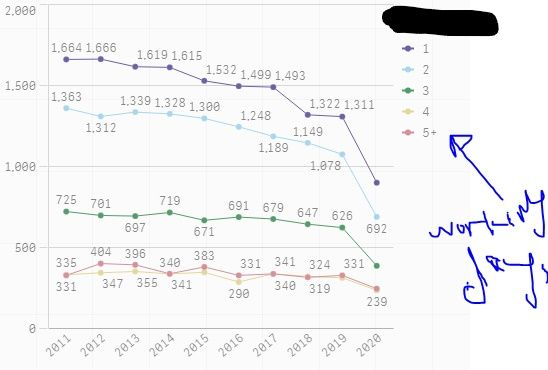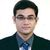# New to Qlik Sense

If you’re new to Qlik Sense, start with this Discussion Board and get up-to-speed quickly.

Announcements
Customer & Partners, DEC. 9, 11 AM ET: Qlik Product & Strategy Roadmap Session: Data Analytics REGISTER NOW
cancel
Showing results for
Did you mean:Contributor III

## Calculate dimension

I created Calculated Dimension in QlikSense , but I got error message for Invalid Dimension. I am trying to create a Line chart that represent the number of projects by working days by year, so:

1- My first dimension is working year

2- My second dimension is an expression to get the numbers of working days by year, but it's not working not sure why? Here is the expression

= If(count(Distinct WorkingDate) = 1, '1',
If(count(Distinct WorkingDate) = 2, '2',
If(count(Distinct WorkingDate) = 3, '3',
If(count(Distinct WorkingDate) = 4, '4',
'+5'))))

3- My Measures is the Count of ProjectId

so I should have a chart that looks like this:1 Solution

Accepted SolutionsMVP

try this.

aggr(If(count(Distinct If(IsPrepDate =0,WorkingDate)) = 1, '1',
If(count(Distinct If(IsPrepDate =0,WorkingDate))= 2, '2',
If(count(Distinct If(IsPrepDate =0,WorkingDate)) = 3, '3',
If(count(Distinct If(IsPrepDate =0,WorkingDate)) = 4, '4',
'+5')))),ProjectId)

Regards,
Kaushik
4 RepliesMVP

Enclosed the expression in another expression called aggr, like below.

=aggr(If(count(Distinct WorkingDate) = 1, '1',
If(count(Distinct WorkingDate) = 2, '2',
If(count(Distinct WorkingDate) = 3, '3',
If(count(Distinct WorkingDate) = 4, '4',
'+5')))),ProjectId)

Regards,
KaushikContributor III
Author

Awesome, thank you so much.  I also want to add a new condition to this expression by counting the distinct work days only if the prepDate is equal to zero, I tried to do it in this way but is not giving the right answer:

=aggr(If(IsPrepDate= 0 and count(Distinct WorkingDate) = 1, '1',
If(IsPrepDate= 0 and count(Distinct WorkingDate) = 2, '2',
If(IsPrepDate= 0 and count(Distinct WorkingDate) = 3, '3',
If(IsPrepDate= 0 and count(Distinct WorkingDate) = 4, '4',
'+5')))),ProjectId)MVP

try this.

aggr(If(count(Distinct If(IsPrepDate =0,WorkingDate)) = 1, '1',
If(count(Distinct If(IsPrepDate =0,WorkingDate))= 2, '2',
If(count(Distinct If(IsPrepDate =0,WorkingDate)) = 3, '3',
If(count(Distinct If(IsPrepDate =0,WorkingDate)) = 4, '4',
'+5')))),ProjectId)

Regards,
Kaushik